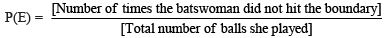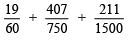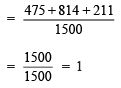Courses

# Ex 15.1 NCERT Solutions- Probability Class 9 Notes | EduRev

## Class 9 Mathematics by Full Circle

Created by: Full Circle

## Class 9 : Ex 15.1 NCERT Solutions- Probability Class 9 Notes | EduRev

The document Ex 15.1 NCERT Solutions- Probability Class 9 Notes | EduRev is a part of the Class 9 Course Class 9 Mathematics by Full Circle.
All you need of Class 9 at this link: Class 9

Question 1. In a cricket match, a batswoman hits a boundary 6 times out of 30 balls she plays. Find the probability that she did not hit a boundary.
Solution: Here, the total number of trials = 30
∵ Number of times the ball touched boundary = 6
∴ Number of times, the ball missed the boundary = 30 – 6 = 24
Let the event not hitting the boundary be represented by E, then= (24/30) = (4/5) = 0.8
Thus, the required probability = 0.8

Question 2. 1500 families with 2 children were selected randomly, and the following data were recorded:

 Number of girls in a family 2 1 0 Number of families 475 814 211

Compute the probability of a family, chosen at random, having
(i) 2 girls
(ii) 1 girl
(iii) No girl
Also check whether the sum of these probabilities is 1.
Solution: Total number of families = 1500.
(i) ∵ Number of families having 2 girls in a family = 475
∴ Probability of a family having 2 girls in a family = (475/1500)= (19/60)

(ii) ∵ Number of families having 1 girl = 814
∴ Probability of a family having 1 girl in a family = (814/1500) = (407/750)

(iii) ∵ Number of families having no girl in a family = 211
∴ Probability of a family having no girl in a family = (211/1500)
Now, the sum of the obtained probabilities
=i.e., Sum of the above probabilities is 1.

Question 3. Refer to Question. Find the probability that a student of the class was born in August.
Solution: From the graph, we have:
Total number of students born in various months in a year = 40
Number of students born in August = 6
∴ Probability of a student of the IX-Class who was born in August = (6/40)= (3/20)

Question 4. Three coins are tossed simultaneously 200 times with the following frequencies of different outcomes:

If the three coins are simultaneously tossed again, compute the probability of 2 heads coming up.
Solution: Total number of times the three coins are tossed = 200
Number of outcomes in which 2 heads coming up = 72
∴ Probability of 2 heads coming up = (72/200) = (9/25)
Thus, the required probability = (9/25)
If the three coins are simultaneously tossed again, then the probability is (9/25)

Question 5. An organisation selected 2400 families at random and surveyed them to determine a relationship between income level and the number of vehicles in a family. The information gathered is listed in the table below:

 Monthly incomeOn ₹) Vehicles per family 0 1 2 Above 2 Less than 7000 10 160 25 0 7000-10000 0 305 27 2 10000-13000 1 535 29 1 13000-16000 2 469 59 25 16000 or more 1 579 82 88
Suppose a family is chosen. Find the probability that the family chosen is
(i) earning  10000–13000 per month and owning exactly 2 vehicles.
(ii) earning  16000 or more per month and owning exactly 1 vehicle.
(iii) earning less than  7000 per month and does not own any vehicle.
(iv) earning  13000–16000 per month and owning more than 2 vehicles.
(v) owning not more than 1 vehicle.
Solution: Here, total number of families = 2400
(i) ∵ Number of families having earning ₹ 10000– ₹ 13000 per month and 2 vehicles = 29
∴ Probability of a family (having earning ₹ 10000–13000 and 2 vehicles) = (29/2400)

(ii) ∵ Number of families having earning ₹16000 or above and owning 1 vehicle = 579
∴ Probability of a family (having earning ₹ 16000 and above and 1 vehicle) = (579/2400)

(iii) ∵ Number of families having earning less than ₹7000 and does not own any vehicle = 10
∴ Probability of a family (having earning less than ₹ 7000 and owning no vehicle) = (10/2400) = (1/240)

(iv) ∵ Number of families having earning ₹13000–16000 and owing more than 2 vehicles = 25
∴ Probability of a family (having earning ₹13000–10000 and owning no vehicle) = (25/2400) = (1/96)

(v) ∵ Number of families owning not more than 1 vehicle
= [Number of families having no vehicle] + [Number of families having only 1 vehicle]
= [10 + 1 + 2+ 1] + [160 + 305 + 535 + 469 + 579]
= 14 + 2148
= 2162
∴ Probability of a family (owning not more than one vehicle) = (2162/2400)
= (1031/1200)

Question 6. Refer to Table.
(i) Find the probability that a student obtained less than 20% in Mathematics test.
(ii) Find the probability that a student obtained 60 marks or above.
Solution:
From the table 14.7, we have:

 Marks Number of students 0–20 20–30 30–40 40–5050–60 60–70 70 and above 710102020158 Total 90

Total number of students = 90
(i) From the given table number of students who have obtained less than 20% marks = 7
⇒ Probability of a student (obtaining less than 20% marks) = (7/90)
(ii) From the given table, number of students who obtained marks 60% or above
= [Number of students in class-interval 60–70] + [Number of students in the class interval 70   and above]
= 15 + 8 = 23
⇒ Probability of a student (who obtained 60 marks and above) = (23/90)

Question 7. To know the opinion of the students about the subject statistics, a survey of 200 students was conducted. The data is recorded in the following table.

 Opinion Number of students Like  Dislike 13565

Find the probability that a student chosen at random (i) likes statistics, (ii) does not like it.
Solution: Total number of students whose opinion in obtained = 200
(i) ∵ Number of students who like statistics = 135
∴ Probability of a student (who likes statistics) = (135/200)
= (27/40)

(ii) ∵ Number of students who do not like statistics = 65
∴ Probability of a student (who dislike statistics) = (65/200) = (13/40)

Question 8.  What is the empirical probability that an engineer lives:
(i) less than 7 km from her place of work?
(ii) More than or equal to 7 km from her place of work?
(iii) Within (1/2) km from her place of work?
Solution: Total number of engineers = 40
(i) ∵ Number of engineers who are living within less than 7 km from their work place = 9
∴ Probability of an engineer living within 7 km from work place = (9/40)

(ii) ∵ Number of engineers living at a distance more than or equal to 7 km from their work place = 31
∴ Probability of an engineer living at a distance more than or equal to 7 km = (31/40)

(iii) ∵ The number of engineers living within (1/2) km from their work place = 0
∴ Probability of an engineer who is living within (1/2) km from work place = (0/40) = 0

Question 9. Activity: Note the frequency of two-wheelers, three-wheelers and four-wheelers going past during a time interval, in front of your school gate. Find the probability that any one vehicle out of the total vehicles you have observed is a two-wheeler.
Solution: It is an activity. Students can do it themselves.

Question 10. Activity: Ask all the students in your class to write a 3-digit number. Choose any student from the room at random. What is the probability that the number written by her/him is divisible by 3?
Solution: A class room activity for students.
REMEMBER
A number is divisible by 3, if the sum of its digits is divisible by 3.
Examples:
(i) Number 45678 is divisible by 3 because 4 + 5 + 6 + 7 + 8 = 30 is divisible by 3
(ii) Number 10786 is not divisible by 3 because 1 + 0 + 7 + 8 + 6 = 22 is not divisible by 3.

Question 11. Eleven bags of wheat flour, each marked 5 kg, actually contained the following weights of flour (in kg):
4.97 5.05 5.08 5.03 5.00 5.06 5.08 4.98 5.04 5.07 5.00

Find the probability that any of these bags chosen at random contains more than 5 kg of flour.
Solution: Total number of bags = 11
∵ Number of bags having more than 5 kg of flour = 7
∴ Probability of a bag (Having more than 5 kg wheat flour) = (7/11)

Question 12. In  this question you were asked to prepare a frequency distribution table, regarding the concentration of sulphur dioxide in the air in parts per million of a certain city for 30 days. Using this table, find the probability of the concentration of sulphur dioxide in the interval 0.12–0.16 on any of these days.
Solution: Total number of days = 30
∵ The number of days (on which the sulphur dioxide concentration is in the interval 0.12–0.16) = 2
∴ Probability of a day (on which sulphur dioxide is in 0.12–0.16 interval) = (2/30)
= (1/15)

Question 13. In  this question, you were asked to prepare a frequency distribution table regarding the blood groups of 30 students of a class. Use this table to determine the probability that a student of this class, selected at random, has blood group AB.
Solution: Total number of students = 30
∵ Number of students having blood group as AB = 3
∴ Probability of a student (whose blood group is AB) = (3/30) = (1/10)

132 docs

,

,

,

,

,

,

,

,

,

,

,

,

,

,

,

,

,

,

,

,

,

;